HashMap面试题，看这一篇就够了！

# 序言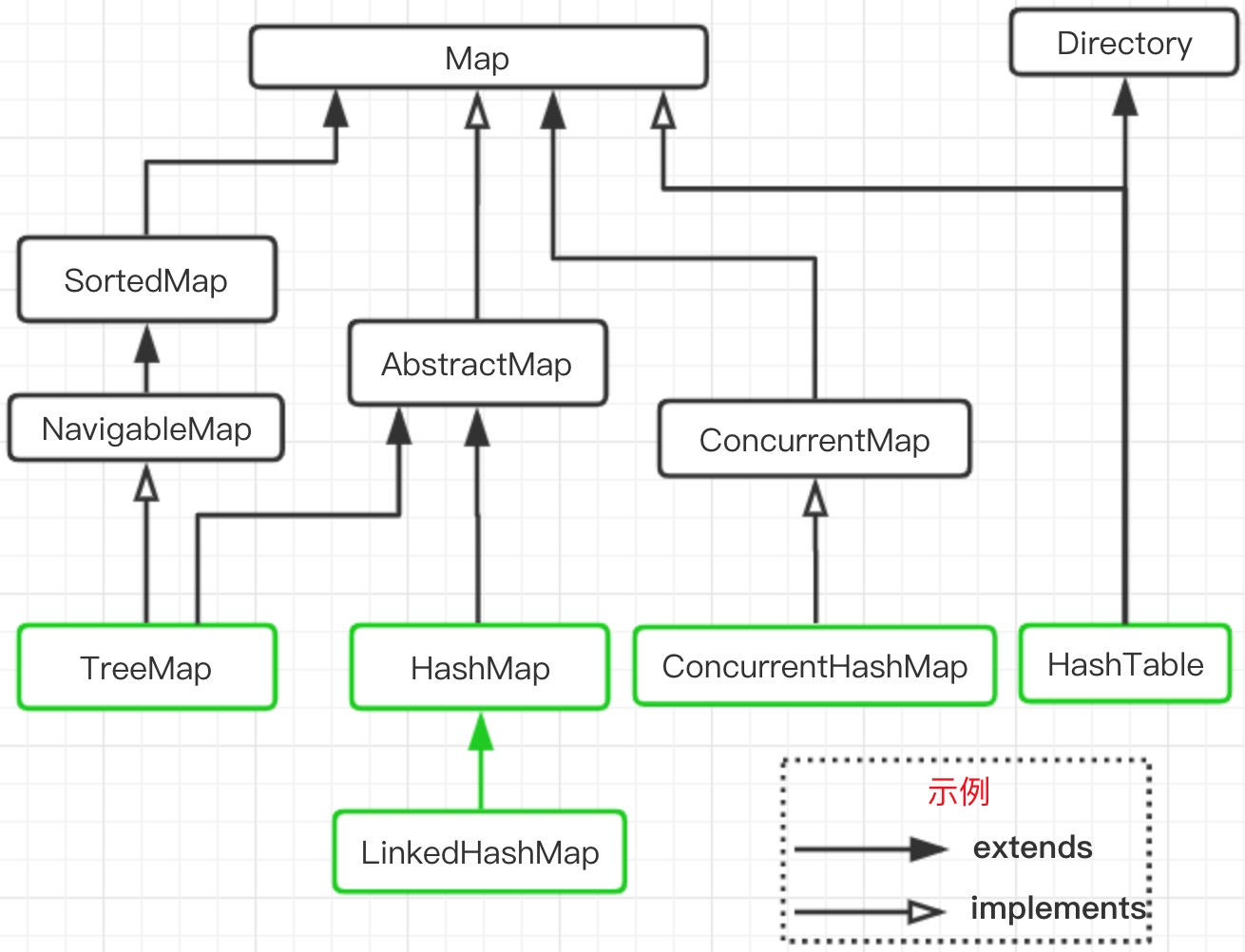HashMap的基本知识点介绍就不多啰嗦了，直奔主题，看JDK7和JDK8的功能实现吧。

# 一、JDK7中的HashMap底层实现

## 1.1 基础知识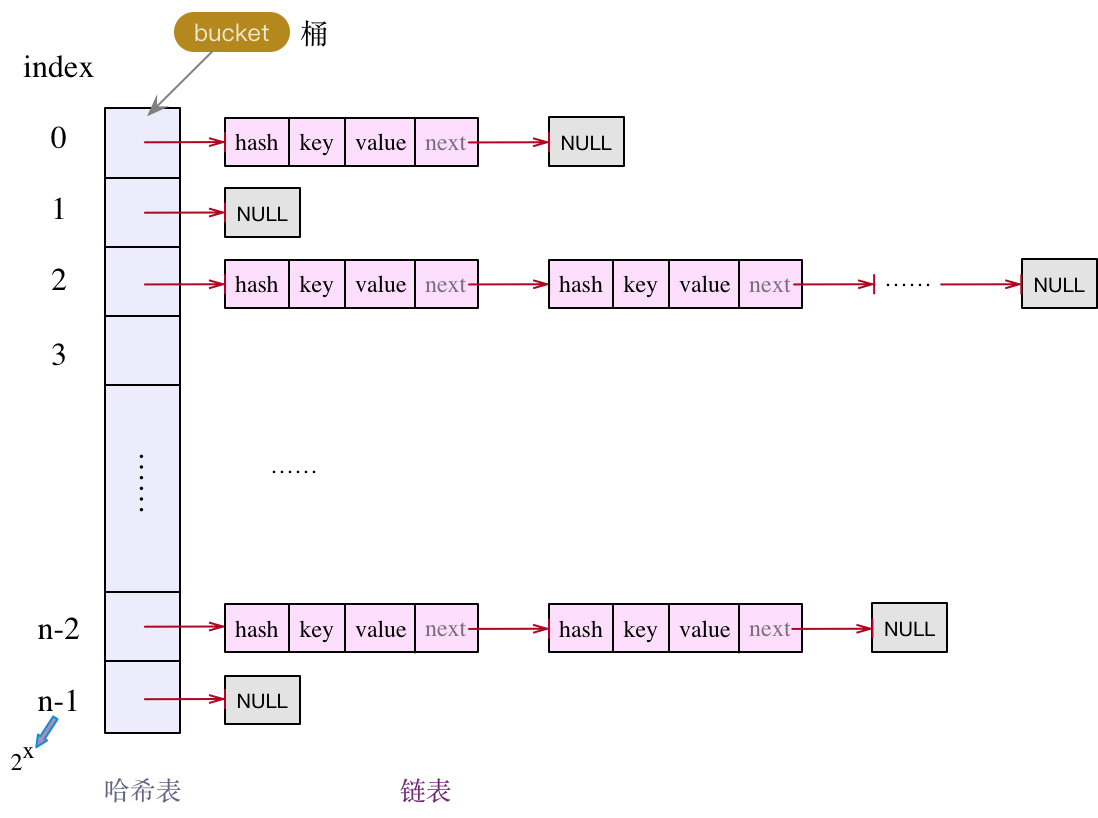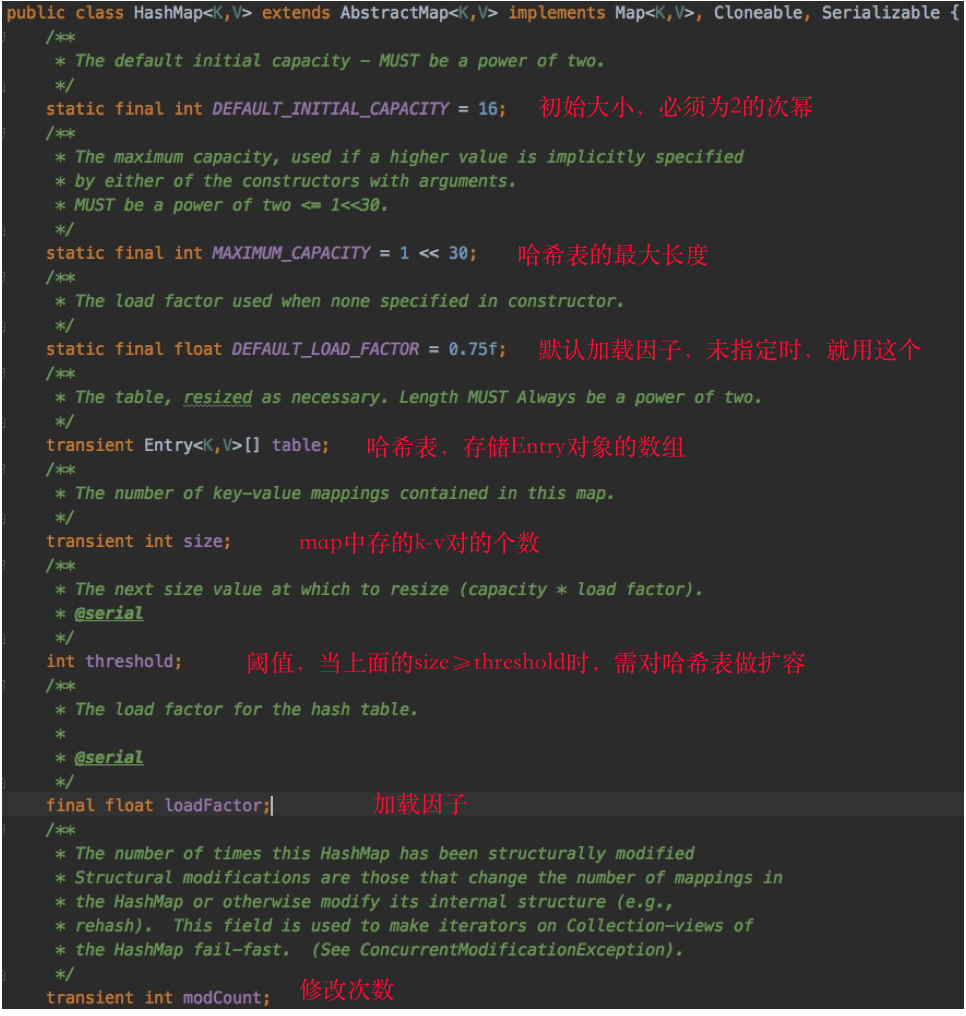```threshold = table.length * loadFactor;
```

## 1.2 put()方法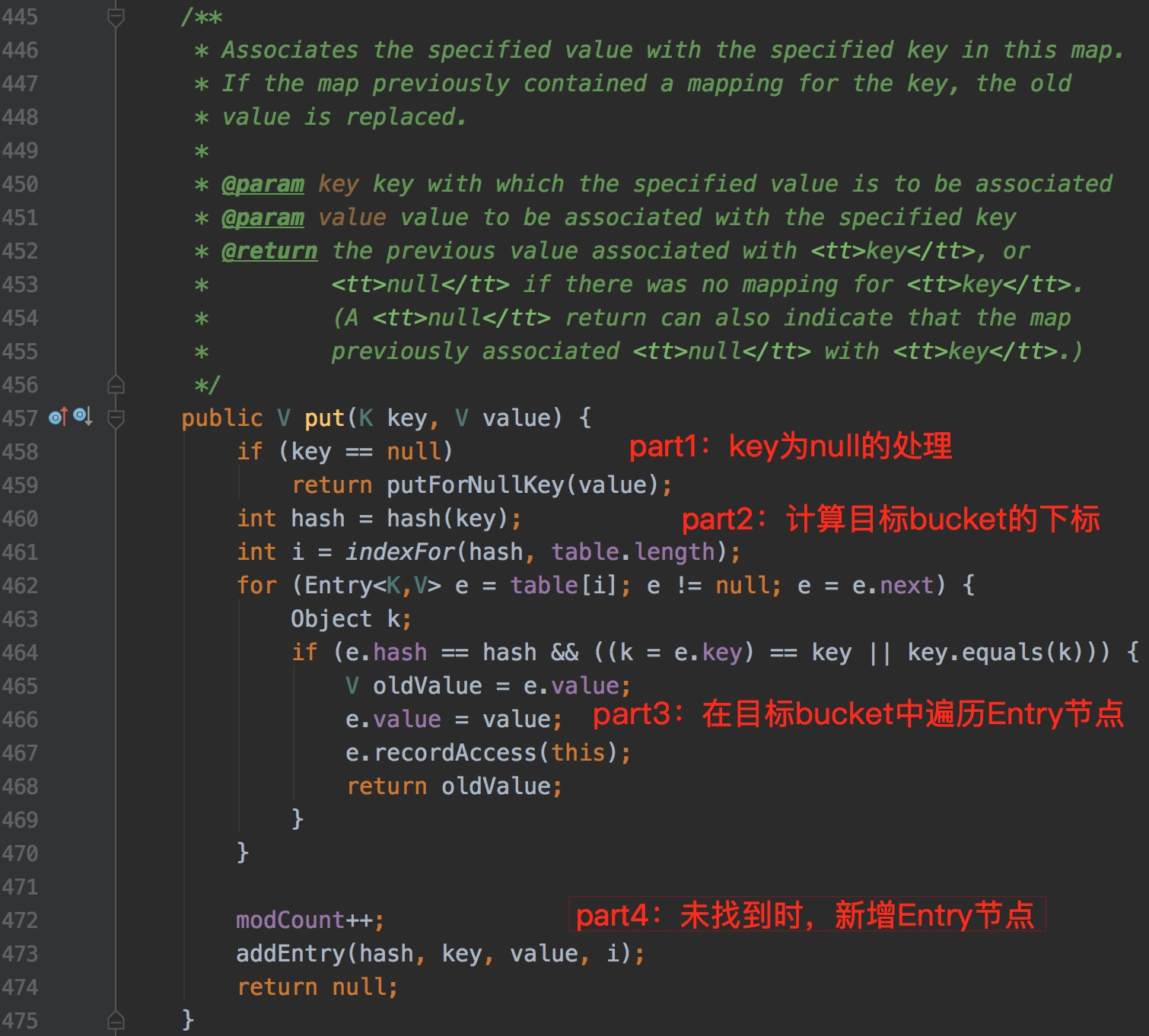• part1：特殊key值处理，key为null；
• part2：计算table中目标bucket的下标；
• part3：指定目标bucket，遍历Entry结点链表，若找到key相同的Entry结点，则做替换；
• part4：若未找到目标Entry结点，则新增一个Entry结点。

• 场景1：若执行put操作前，key已经存在，那么在执行put操作时，会使用本次的新value值来覆盖前一次的旧value值，返回的就是旧value值；
• 场景2：若key不存在，则返回null值。

### 1.2.1 特殊key值处理

a) HashMap中，是允许key、value都为null的，且key为null只存一份，多次存储会将旧value值覆盖；

b) key为null的存储位置，都统一放在下标为0的bucket，即：table位置的链表；

c) 如果是第一次对key=null做put操作，将会在table的位置新增一个Entry结点，使用头插法做链表插入。

```private V putForNullKey(V value) {
for (Entry<K,V> e = table; e != null; e = e.next) {
if (e.key == null) {
V oldValue = e.value;
e.value = value;
e.recordAccess(this);
return oldValue;
}
}
modCount++;
return null;
}

/**
* Adds a new entry with the specified key, value and hash code to
* the specified bucket.  It is the responsibility of this
* method to resize the table if appropriate.
*
* Subclass overrides this to alter the behavior of put method.
*/
void addEntry(int hash, K key, V value, int bucketIndex) {
if ((size >= threshold) && (null != table[bucketIndex])) {
resize(2 * table.length);
hash = (null != key) ? hash(key) : 0;
bucketIndex = indexFor(hash, table.length);
}

createEntry(hash, key, value, bucketIndex);
}

/**
* Like addEntry except that this version is used when creating entries
* as part of Map construction or "pseudo-construction" (cloning,
* deserialization).  This version needn't worry about resizing the table.
*
* Subclass overrides this to alter the behavior of HashMap(Map),
*/
void createEntry(int hash, K key, V value, int bucketIndex) {
Entry<K,V> e = table[bucketIndex];
table[bucketIndex] = new Entry<>(hash, key, value, e);
size++;
}
```

`putForNullKey()`方法中的代码较为简单：首先选择table位置的链表，然后对链表做遍历操作，如果有结点的key为null，则将新value值替换掉旧value值，返回旧value值，如果未找到，则新增一个key为null的Entry结点。

### 1.2.2 扩容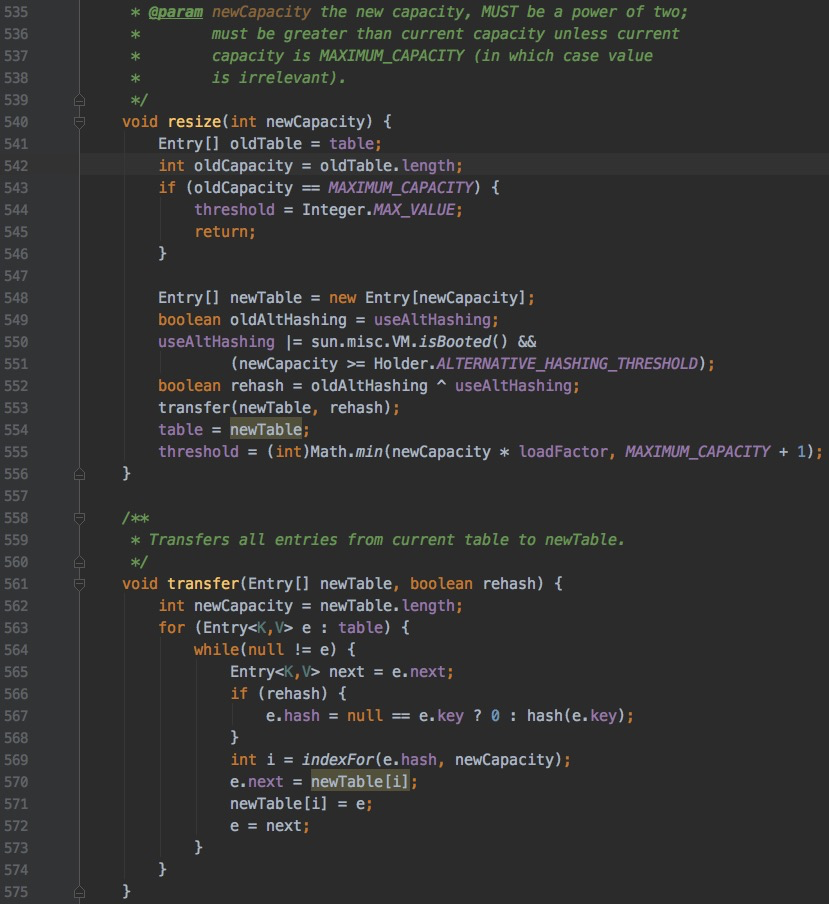a) 扩容后大小是扩容前的2倍；

```oldCapacity=table.length;
newCapacity = 2 * oldCapacity;
```

b) 数据搬迁，从旧table迁到扩容后的新table。

### 1.2.3 如何计算bucket下标？

#### ① hash值的计算

```String str1 = "abc";
String str2 = new String("abc");
System.out.println(str1 == str2);  // false，两个对象的内存地址并不同
System.out.println(str1.equals(str2)); // true 两个对象的域值相同，都存储了 abc 这三个字符
```

```System.out.println(str1.hashCode() == str2.hashCode());

---输出---
true
```

```String str1 = "abc";
String str2 = new String("abc");
Map<String, Integer> testMap = new HashMap<>();
testMap.put(str1, 12);
testMap.put(str2, 13);

String str3 = new StringBuilder("ab").append("c").toString();
System.out.println(testMap.get(str3));

---输出---
13
```

#### ② 取模的逻辑

```/**
* Returns index for hash code h.
*/
static int indexFor(int h, int length) {
return h & (length-1);
}
```

### 1.2.4 在目标bucket中遍历Entry结点

```...
int i = indexFor(hash, table.length);
for (Entry<K,V> e = table[i]; e != null; e = e.next) {
Object k;
if (e.hash == hash && ((k = e.key) == key || key.equals(k))) {
V oldValue = e.value;
e.value = value;
e.recordAccess(this);
return oldValue;
}
}
...
```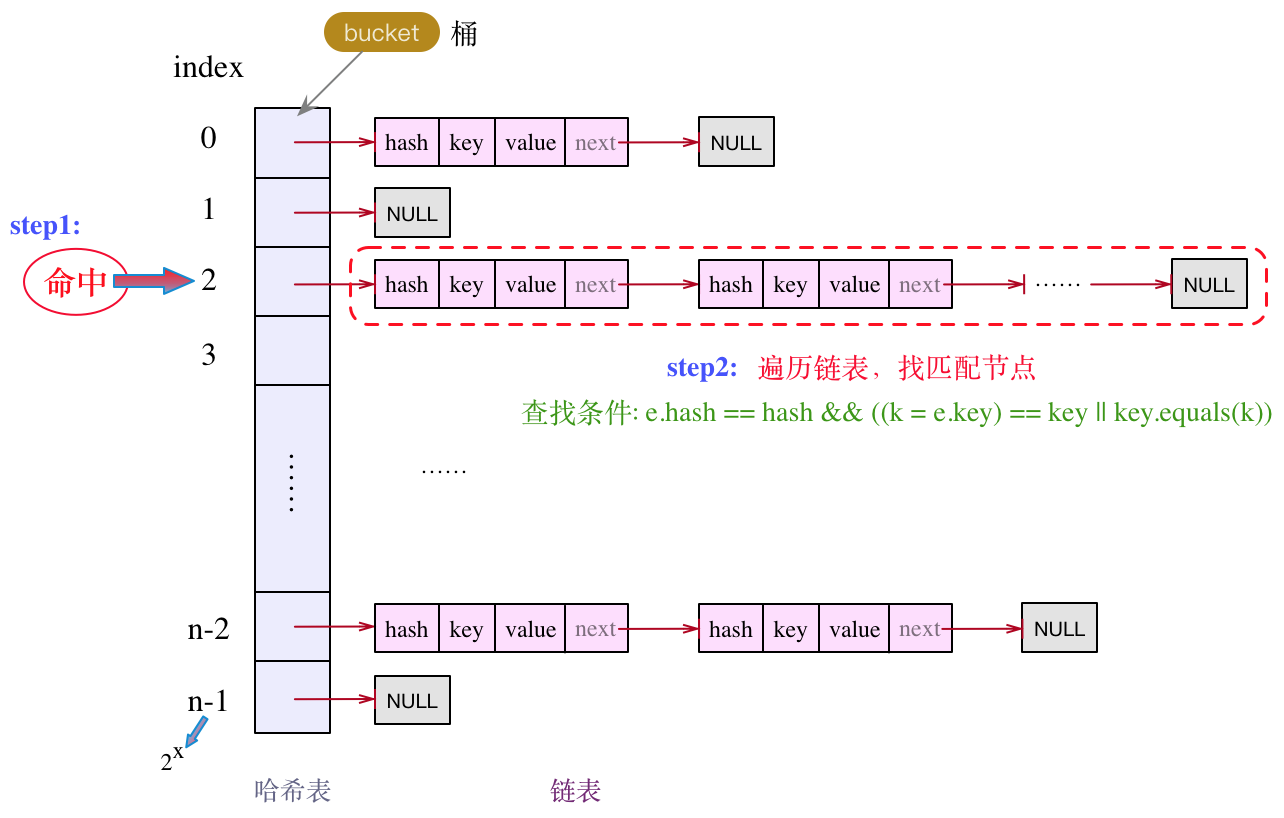## 1.3 get()方法

```public V get(Object key) {
if (key == null)
return getForNullKey();
Entry<K,V> entry = getEntry(key);

return null == entry ? null : entry.getValue();
}

/**
* Returns the entry associated with the specified key in the
* HashMap.  Returns null if the HashMap contains no mapping
* for the key.
*/
final Entry<K,V> getEntry(Object key) {
int hash = (key == null) ? 0 : hash(key);
for (Entry<K,V> e = table[indexFor(hash, table.length)];
e != null;
e = e.next) {
Object k;
if (e.hash == hash &&
((k = e.key) == key || (key != null && key.equals(k))))
return e;
}
return null;
}
```

## 1.4 Map中的迭代器Iterator

### 1.4.1 Map遍历的几种方式

#### 方式1：Iterator迭代器

```Iterator<Entry<String, Integer>> iterator = testMap.entrySet().iterator();
while (iterator.hasNext()) {
Entry<String, Integer> next = iterator.next();
System.out.println(next.getKey() + ":" + next.getValue());
}
```

#### 方式2：最常见的使用方式，可同时得到key、value值

```// 方式一
for (Map.Entry<String, Integer> entry : testMap.entrySet()) {
System.out.println(entry.getKey() + ":" + entry.getValue());
}
```

```Iterator var2 = testMap.entrySet().iterator();
while(var2.hasNext()) {
Entry<String, Integer> entry = (Entry)var2.next();
System.out.println((String)entry.getKey() + ":" + entry.getValue());
}
```

#### 方式3：使用foreach方式（JDK1.8才有）

```testMap.forEach((key, value) -> {
System.out.println(key + ":" + value);
});
```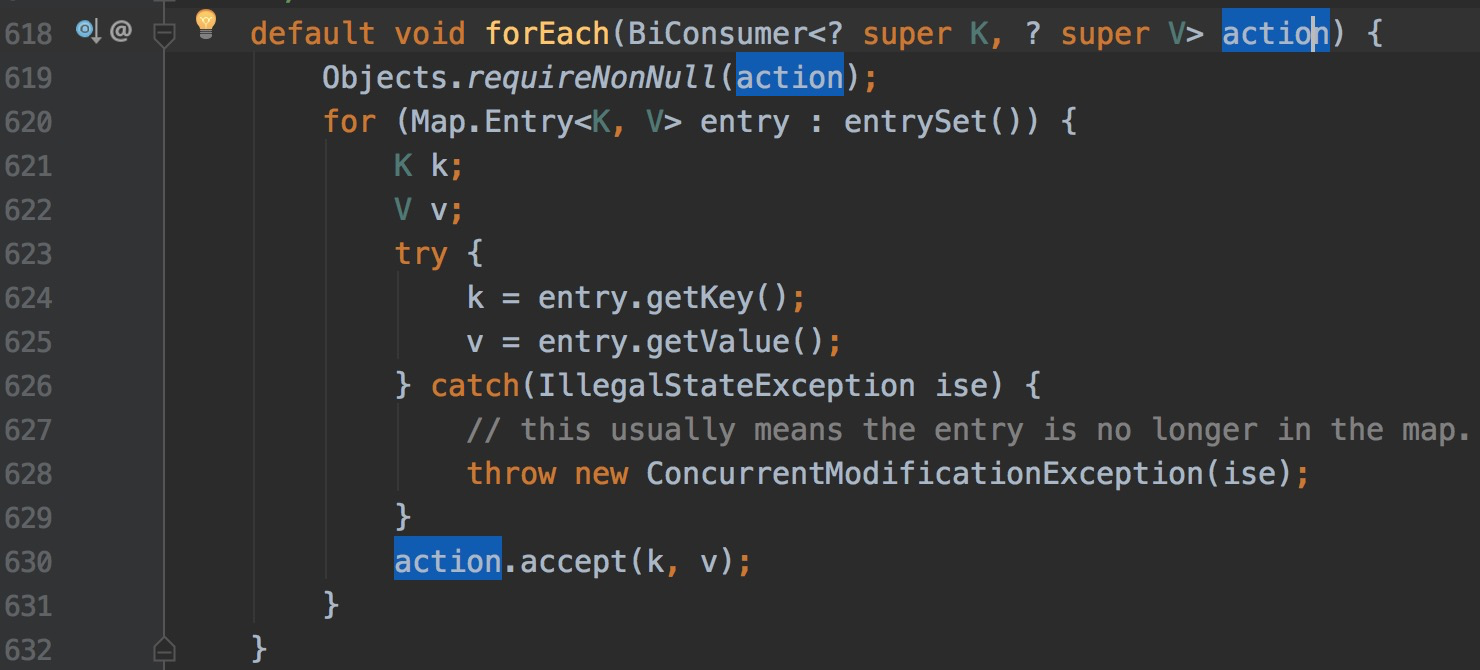#### 方式4：通过key的set集合遍历

```Iterator<String> keyIterator = testMap.keySet().iterator();
while (keyIterator.hasNext()) {
String key = keyIterator.next();
System.out.println(key + ":" + testMap.get(key));
}
```

```for (String key : testMap.keySet()) {
System.out.println(key + ":" + testMap.get(key));
}
```

### 1.4.2 Iterator的实现原理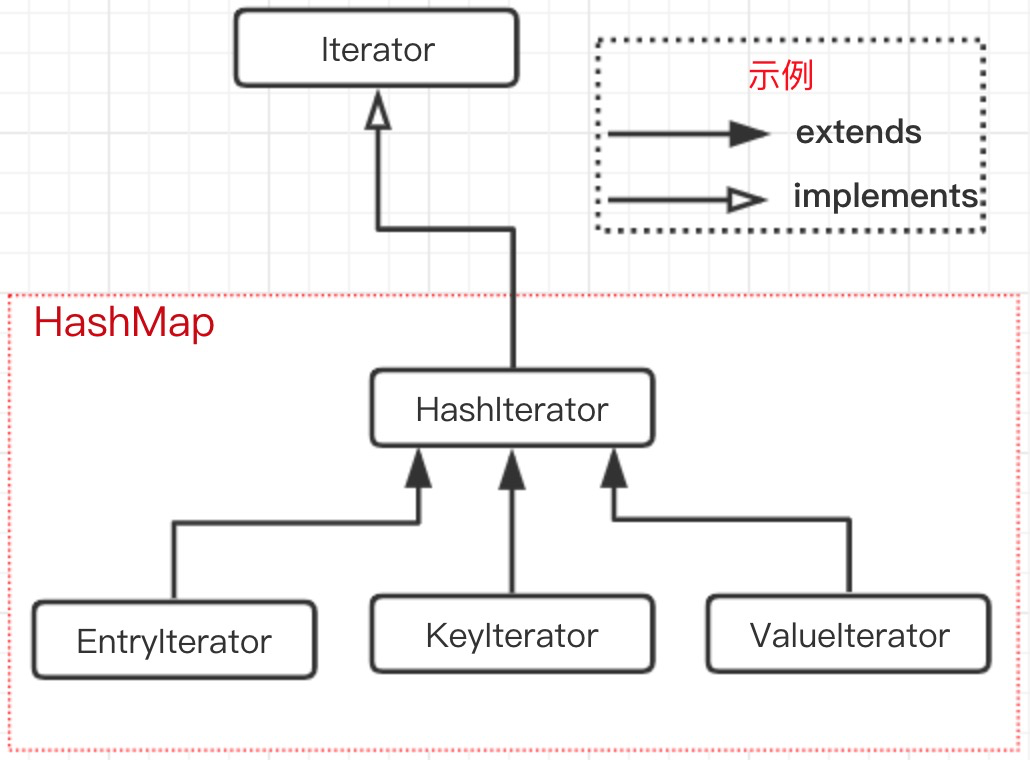Iterator为一个顶层接口，只提供了三个基础方法声明：

```public interface Iterator<E> {

boolean hasNext();

E next();

default void remove() {
throw new UnsupportedOperationException("remove");
}
}
```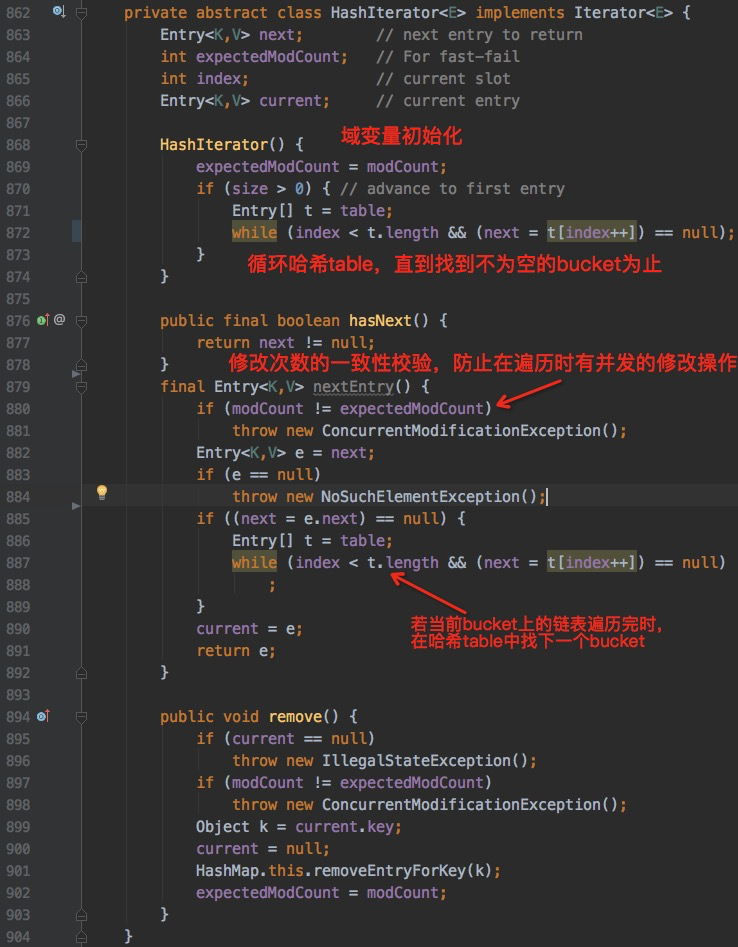```Iterator<Entry<String, Integer>> iterator = testMap.entrySet().iterator();
while (iterator.hasNext()) {
Entry<String, Integer> next = iterator.next();
System.out.println(next.getKey() + ":" + next.getValue());
}
```

```private final class EntryIterator extends HashIterator<Map.Entry<K,V>> {
public Map.Entry<K,V> next() {
return nextEntry();
}
}
```

HashMap中的Iterator实现原理也不过如此，就是这么朴实无华，是不是都想动手自己撸一个HashMap的实现了？嗯，你可以的！！！

## 1.5 fail-fast策略

In systems design, a fail-fast system is one which immediately reports at its interface any condition that is likely to indicate a failure. Fail-fast systems are usually designed to stop normal operation rather than attempt to continue a possibly flawed process.
Such designs often check the system's state at several points in an operation, so any failures can be detected early. The responsibility of a fail-fast module is detecting errors, then letting the next-highest level of the system handle them.

```public static void main(String[] args) {
Map<String, Integer> testMap = new HashMap<>();
testMap.put("s1", 11);
testMap.put("s2", 22);
testMap.put("s3", 33);

for (Map.Entry<String, Integer> entry : testMap.entrySet()) {
String key = entry.getKey();
if ("s1".equals(key)) {
testMap.remove(key);
}
}
}

---- output ---
at java.util.HashMap\$HashIterator.nextNode(HashMap.java:1437)
at java.util.HashMap\$EntryIterator.next(HashMap.java:1471)
at java.util.HashMap\$EntryIterator.next(HashMap.java:1469)
...
```

```// 方式三
Iterator<Entry<String, Integer>> iterator = testMap.entrySet().iterator();
while (iterator.hasNext()) {
Entry<String, Integer> next = iterator.next();
System.out.println(next.getKey() + ":" + next.getValue());
if (next.getKey().equals("s2")) {
iterator.remove();
}
}
```

# 二、JDK8中的HashMap底层实现

JDK8中的HashMap其底层存储结构如下：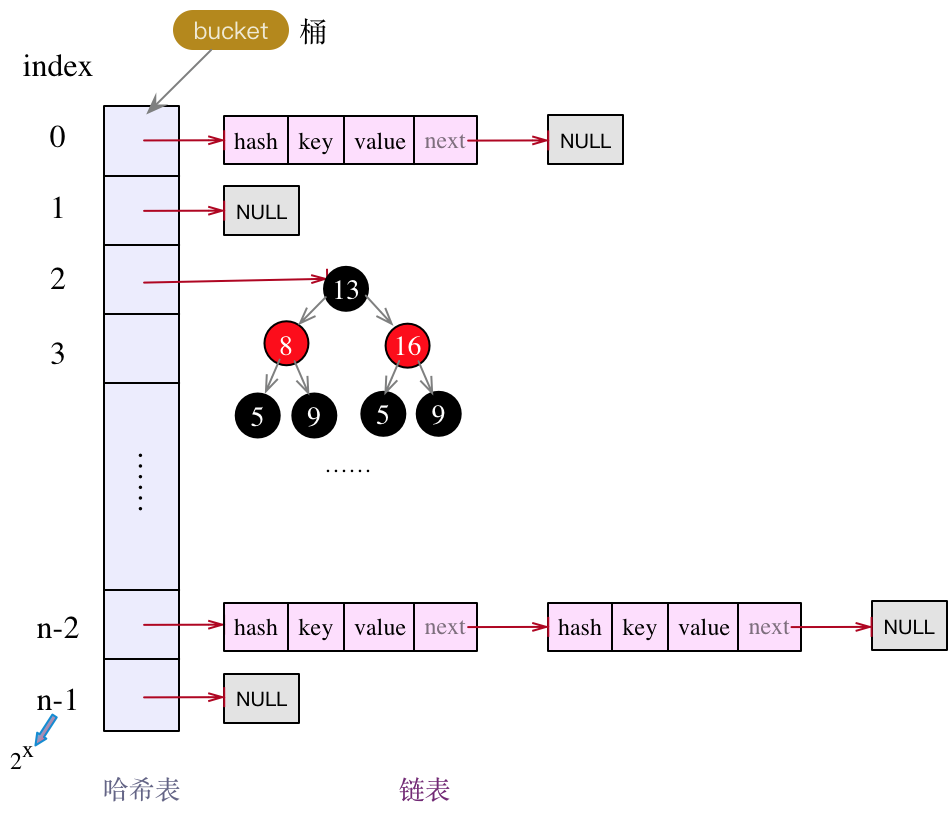## 2.1 put()操作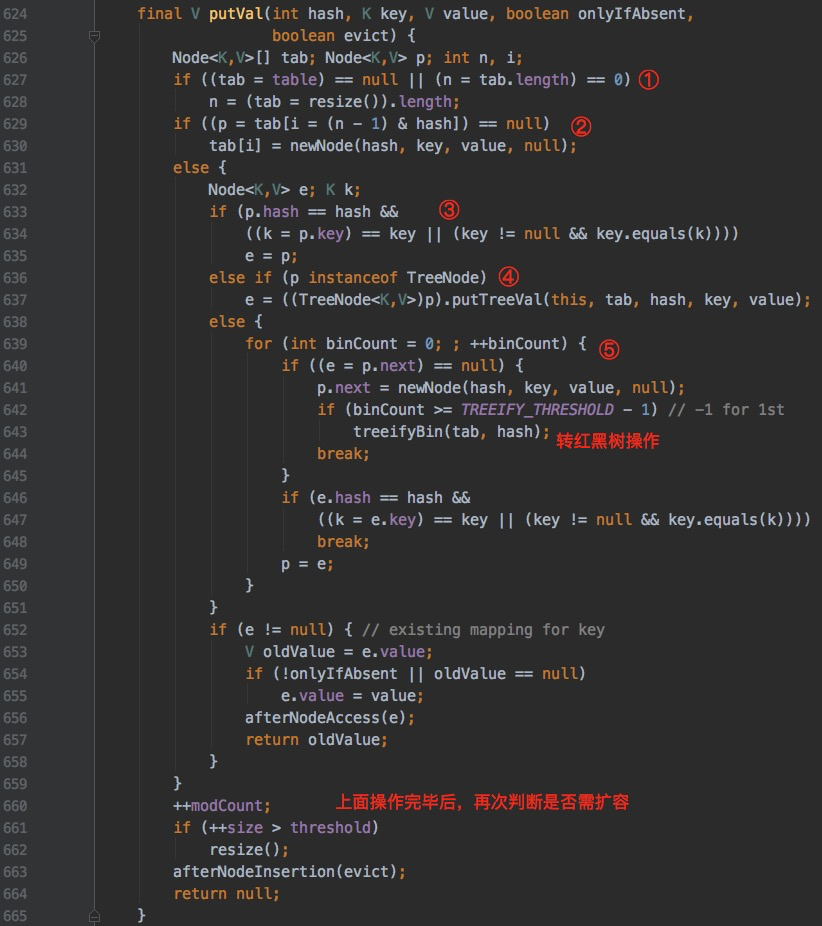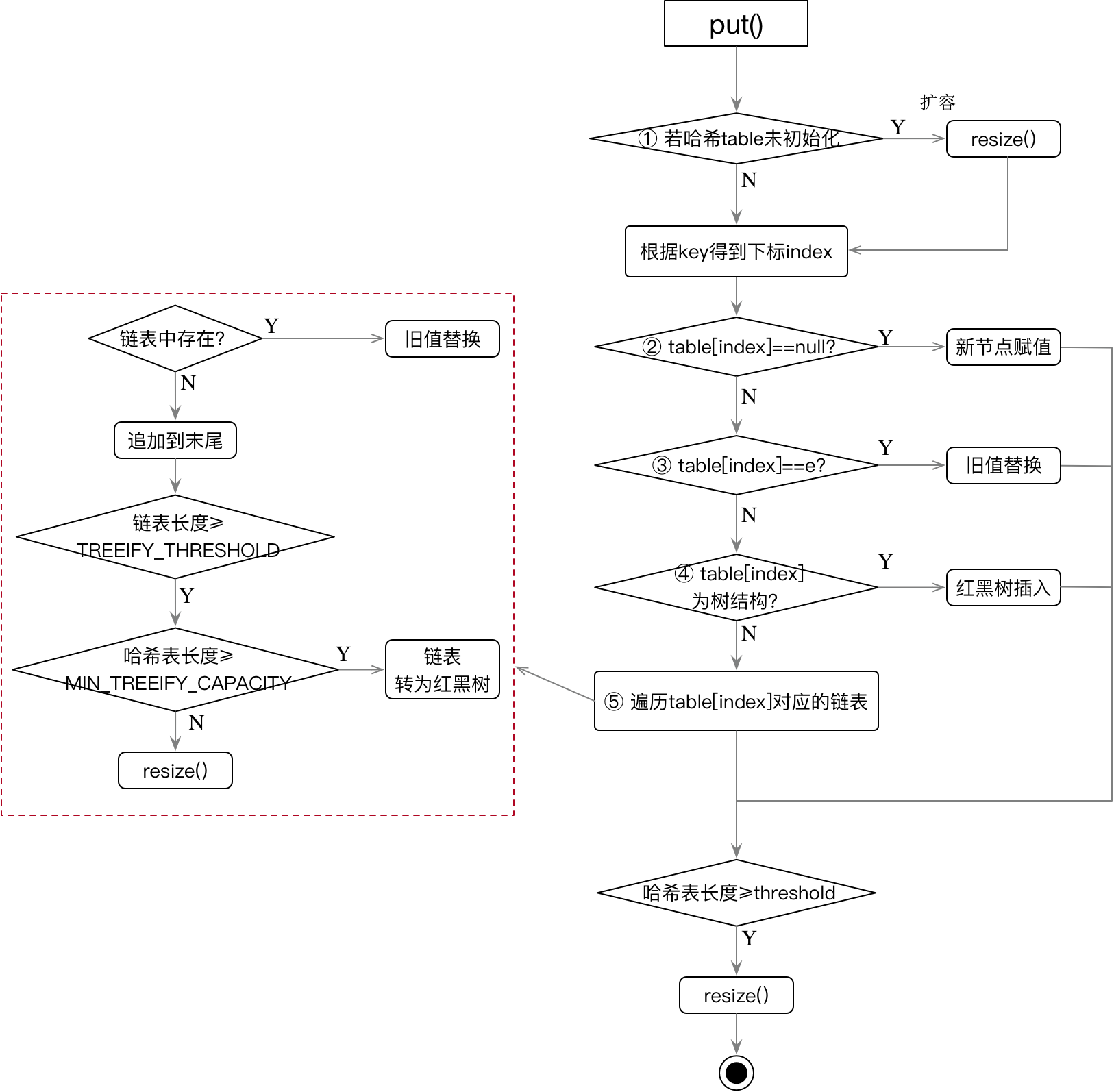a) 若链表中有结点匹配，则做value替换；

b）若没有结点匹配，则在链表末尾追加。同时，执行以下操作：

i) 若链表长度大于`TREEIFY_THRESHOLD`，则执行红黑树转换操作；

ii) 若条件i) 不成立，则执行扩容resize()操作。

```/**
* Replaces all linked nodes in bin at index for given hash unless
* table is too small, in which case resizes instead.
*/
final void treeifyBin(Node<K,V>[] tab, int hash) {
int n, index; Node<K,V> e;
// 若表为空，或表长度小于MIN_TREEIFY_CAPACITY，也不做转换，直接做扩容处理。
if (tab == null || (n = tab.length) < MIN_TREEIFY_CAPACITY)
resize();
else if ((e = tab[index = (n - 1) & hash]) != null) {
TreeNode<K,V> hd = null, tl = null;
do {
TreeNode<K,V> p = replacementTreeNode(e, null);
if (tl == null)
hd = p;
else {
p.prev = tl;
tl.next = p;
}
tl = p;
} while ((e = e.next) != null);
if ((tab[index] = hd) != null)
hd.treeify(tab);
}
}
```

## 2.2 扩容操作

```...
// 前面已经做了第1步的长度拓展，我们主要分析第2步的操作：如何迁移数据
table = newTab;
if (oldTab != null) {
// 循环遍历哈希table的每个不为null的bucket
// 注意，这里是"++j"，略过了oldTab的处理
for (int j = 0; j < oldCap; ++j) {
Node<K,V> e;
if ((e = oldTab[j]) != null) {
oldTab[j] = null;
// 若只有一个结点，则原地存储
if (e.next == null)
newTab[e.hash & (newCap - 1)] = e;
else if (e instanceof TreeNode)
((TreeNode<K,V>)e).split(this, newTab, j, oldCap);
else { // preserve order
// "lo"前缀的代表要在原bucket上存储，"hi"前缀的代表要在新的bucket上存储
Node<K,V> loHead = null, loTail = null;
Node<K,V> hiHead = null, hiTail = null;
Node<K,V> next;
do {
next = e.next;
// 以oldCap=8为例，
//   0001 1000  e.hash=24
// & 0000 1000  oldCap=8
// = 0000 1000  --> 不为0，需要迁移
// 这种规律可发现，[oldCap, (2*oldCap-1)]之间的数据，
// 以及在此基础上加n*2*oldCap的数据，都需要做迁移，剩余的则不用迁移
if ((e.hash & oldCap) == 0) {
// 这种是有序插入，即依次将原链表的结点追加到当前链表的末尾
if (loTail == null)
else
loTail.next = e;
loTail = e;
}
else {
if (hiTail == null)
else
hiTail.next = e;
hiTail = e;
}
} while ((e = next) != null);
if (loTail != null) {
loTail.next = null;
}
if (hiTail != null) {
hiTail.next = null;
// 需要搬迁的结点，新下标为从当前下标往前挪oldCap个距离。
}
}
}
}
}
```

## 2.3 get()操作

```public V get(Object key) {
Node<K,V> e;
return (e = getNode(hash(key), key)) == null ? null : e.value;
}

final Node<K,V> getNode(int hash, Object key) {
Node<K,V>[] tab; Node<K,V> first, e; int n; K k;
if ((tab = table) != null && (n = tab.length) > 0 &&
(first = tab[(n - 1) & hash]) != null) {
if (first.hash == hash && // always check first node
((k = first.key) == key || (key != null && key.equals(k))))
return first;
if ((e = first.next) != null) {
if (first instanceof TreeNode)
return ((TreeNode<K,V>)first).getTreeNode(hash, key);
do {
if (e.hash == hash &&
((k = e.key) == key || (key != null && key.equals(k))))
return e;
} while ((e = e.next) != null);
}
}
return null;
}
```

# 三、HashMap、HashTable是什么关系？## 3.1 共同点与异同点

• 底层都是使用哈希表 + 链表的实现方式。

• 从层级结构上看，HashMap、HashTable有一个共用的`Map`接口。另外，HashTable还单独继承了一个抽象类`Dictionary`
• HashTable诞生自JDK1.0，HashMap从JDK1.2之后才有；
• HashTable线程安全，HashMap线程不安全；
• 初始值和扩容方式不同。HashTable的初始值为11，扩容为原大小的`2*d+1`。容量大小都采用奇数且为素数，且采用取模法，这种方式散列更均匀。但有个缺点就是对素数取模的性能较低（涉及到除法运算），而HashMap的长度都是2的次幂，设计就较为巧妙，前面章节也提到过，这种方式的取模都是直接做位运算，性能较好。
• HashMap的key、value都可为null，且value可多次为null，key多次为null时会覆盖。当HashTable的key、value都不可为null，否则直接NPE(NullPointException)。

```public static void main(String[] args) {
Map<String, Integer> testTable = new Hashtable<>();
testTable.put(null, 23);  // 抛NPE
testTable.put("s1", null); // 抛NPE
}
```

```public synchronized V put(K key, V value) {
// Make sure the value is not null
if (value == null) {
throw new NullPointerException();
}

// Makes sure the key is not already in the hashtable.
Entry<?,?> tab[] = table;
int hash = key.hashCode();
int index = (hash & 0x7FFFFFFF) % tab.length;
@SuppressWarnings("unchecked")
Entry<K,V> entry = (Entry<K,V>)tab[index];
for(; entry != null ; entry = entry.next) {
if ((entry.hash == hash) && entry.key.equals(key)) {
V old = entry.value;
entry.value = value;
return old;
}
}

return null;
}
```

```<strong>NOTE: This class is obsolete.  New implementations should
implement the Map interface, rather than extending this class.</strong>
```

## 3.2 HashMap的线程安全

put操作、get操作、remove操作、equals操作，都使用了`synchronized`关键字修饰。

# 四、HashMap线程不安全在哪？

## 4.1 数据覆盖问题

```public V put(K key, V value) {
...
}

void addEntry(int hash, K key, V value, int bucketIndex) {
...
createEntry(hash, key, value, bucketIndex);
}

void createEntry(int hash, K key, V value, int bucketIndex) {
Entry<K,V> e = table[bucketIndex];
table[bucketIndex] = new Entry<>(hash, key, value, e);
size++;
}
```

## 4.2 扩容时导致死循环

```void transfer(Entry[] newTable, boolean rehash) {
int newCapacity = newTable.length;
for (Entry<K,V> e : table) {
while(null != e) {
Entry<K,V> next = e.next;
if (rehash) {
e.hash = null == e.key ? 0 : hash(e.key);
}
int i = indexFor(e.hash, newCapacity);
e.next = newTable[i];
newTable[i] = e;
e = next;
}
}
}
```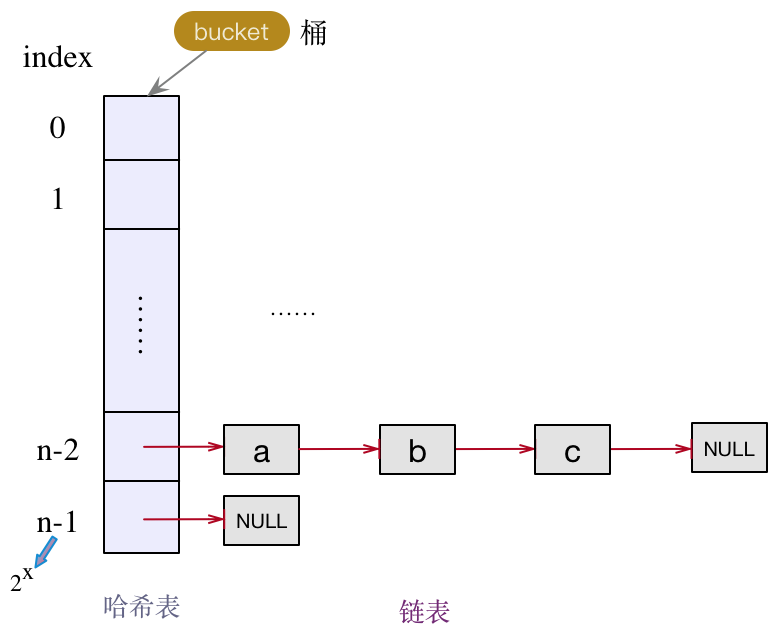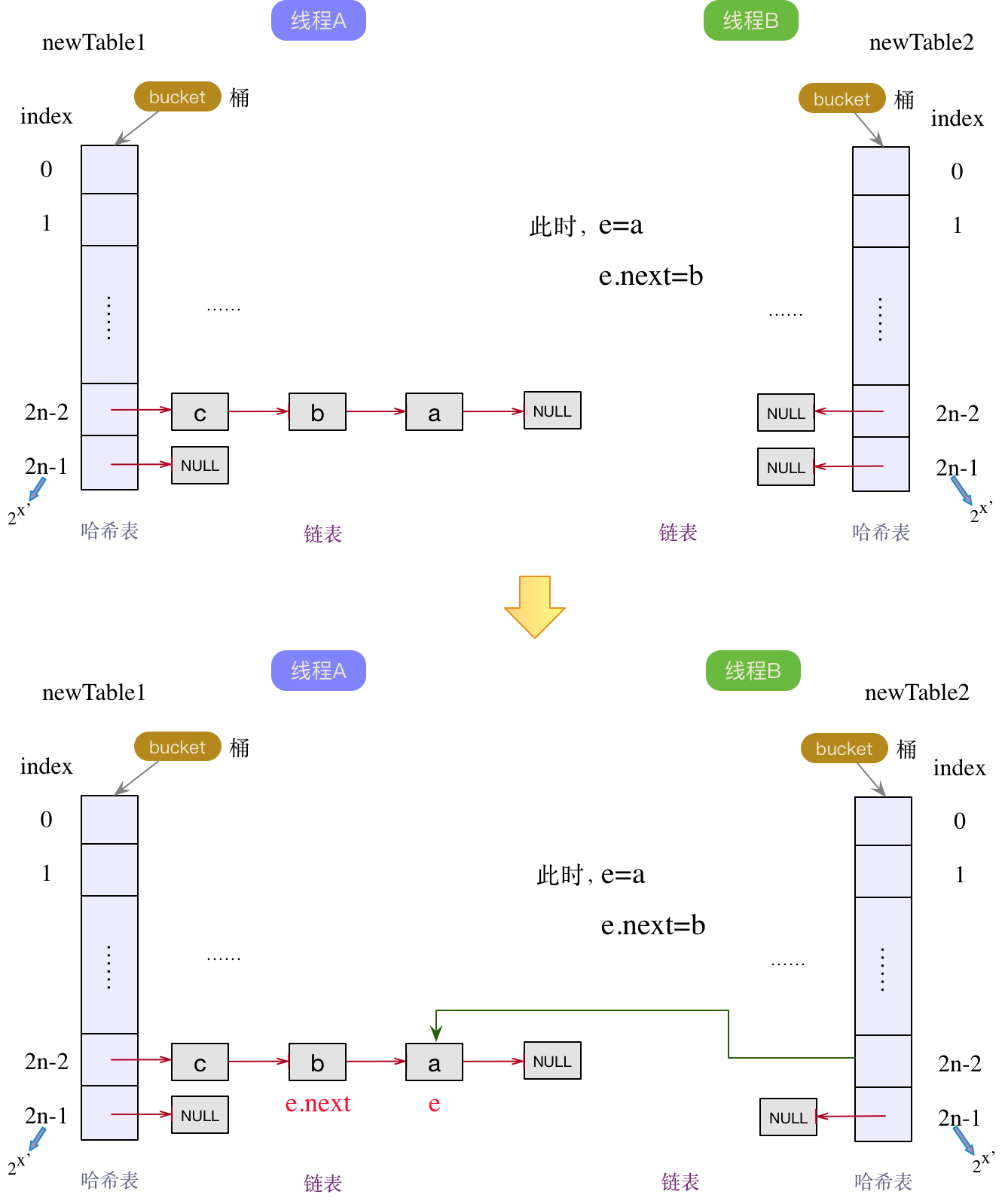a结点成为线程B中链表的头结点后，下一个结点`e.next`为b结点。既然下一个结点`e.next`不为null，那么当前结点`e`就变成了b结点，下一个结点`e.next`变为a结点。继续执行头插法，将b变为链表的头结点，同时next指针指向旧的头节点a，如下图：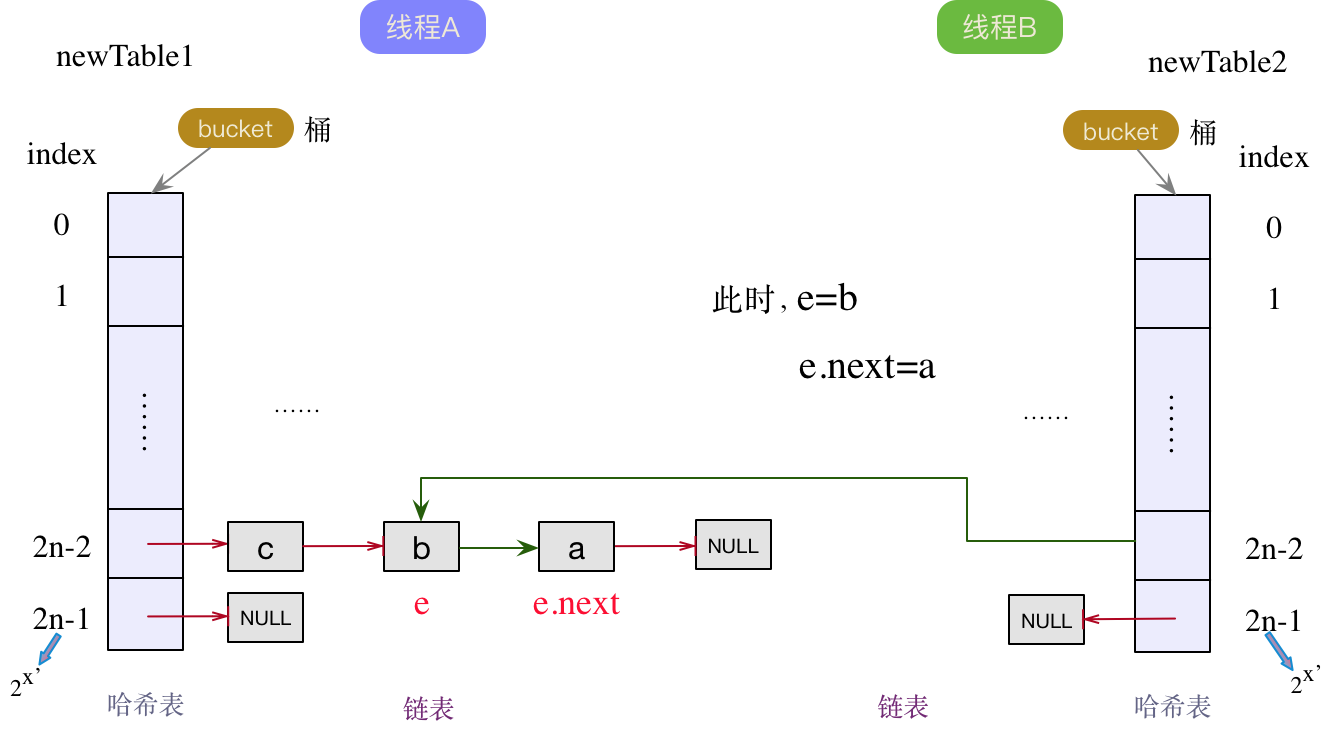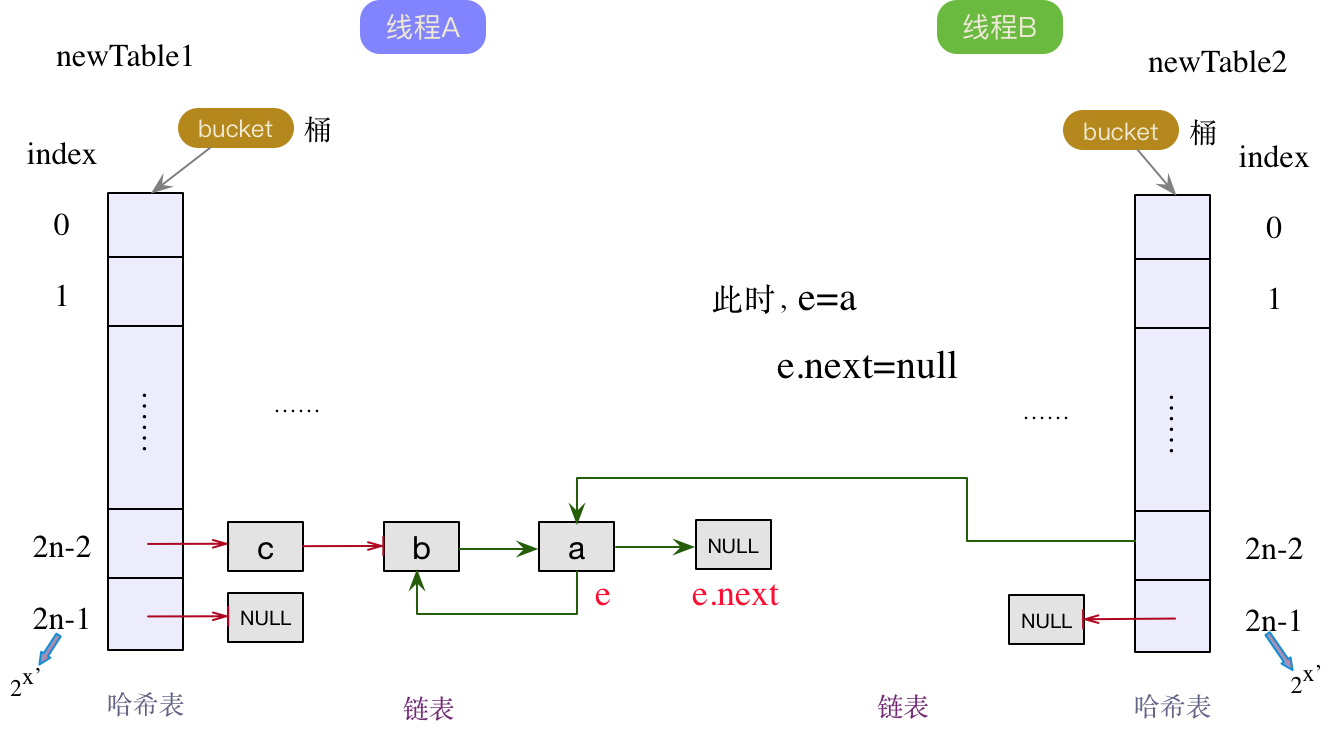• 死循环
• 数据重复
• 数据丢失（覆盖）

# 五、如何规避HashMap的线程不安全？

## 5.1 将Map转为包装类

```Map<String, Integer> testMap = new HashMap<>();
...
// 转为线程安全的map
Map<String, Integer> map = Collections.synchronizedMap(testMap);
```

```// 源码来自Collections类

private static class SynchronizedMap<K,V> implements Map<K,V>, Serializable {
private static final long serialVersionUID = 1978198479659022715L;

private final Map<K,V> m;     // Backing Map
final Object      mutex;        // Object on which to synchronize

SynchronizedMap(Map<K,V> m) {
this.m = Objects.requireNonNull(m);
mutex = this;
}

SynchronizedMap(Map<K,V> m, Object mutex) {
this.m = m;
this.mutex = mutex;
}

public int size() {
synchronized (mutex) {return m.size();}
}
public boolean isEmpty() {
synchronized (mutex) {return m.isEmpty();}
}
public boolean containsKey(Object key) {
synchronized (mutex) {return m.containsKey(key);}
}
public boolean containsValue(Object value) {
synchronized (mutex) {return m.containsValue(value);}
}
public V get(Object key) {
synchronized (mutex) {return m.get(key);}
}

public V put(K key, V value) {
synchronized (mutex) {return m.put(key, value);}
}
public V remove(Object key) {
synchronized (mutex) {return m.remove(key);}
}
public void putAll(Map<? extends K, ? extends V> map) {
synchronized (mutex) {m.putAll(map);}
}
public void clear() {
synchronized (mutex) {m.clear();}
}
}
```

## 5.2 使用ConcurrentHashMap

```Map<String, Integer> susuMap = new ConcurrentHashMap<>();
susuMap.put("susu1", 111);
susuMap.put("susu2", 222);
System.out.println(susuMap.get("susu1"));
```

JDK1.5版本引入，位于并发包`java.util.concurrent`下。